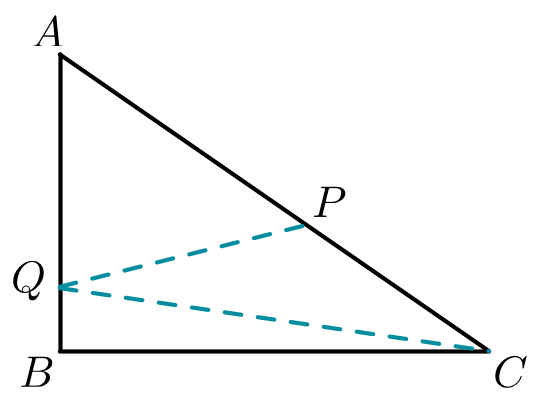# Line from nowhere

Geometry Level 4Let $ABC$ be a right triangle with $\angle ABC=90^\circ$. Let $P$ be a point on segment $AC$ such that $\frac{AP}{PC}=\frac{3}{2}$ and let $Q$ be a point on segment $AB$ such that $AQ=3\cdot QB$. If $\angle AQP=2 \angle PQC$, what is the measure (in degrees) of $\angle PQC$ ?

×

Problem Loading...

Note Loading...

Set Loading...